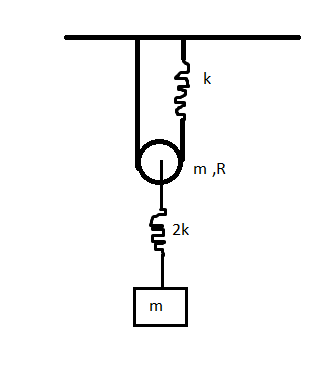# Just hanging around - part3If the time period of SHM of rectangular block can be represented as $T=2\pi \sqrt{\frac{m(\sqrt a +b)}{ck}}$

Find the value of $a+b+c$.

Details and Assumptions:

• Treat the pulley as a disc.

• The block and pulley are oscillating in the same phase with the same frequency.

• Pulley has sufficient friction for Pure Rolling and mass=m & strings are light and inextensible.

• Gravity is present.

• Zig-zag lines in fig represents spring of spring constant $k$ and $2k$ as shown.

• a is a square-free integer and the fraction is in simplest form.

×